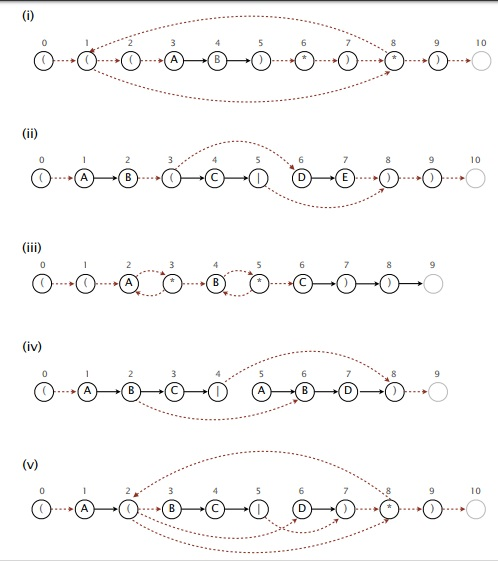### REGULAR EXPRESSIONS STUDY GUIDE

Terminology and Basics

• Understand the regular expression syntax.
• Know how to write simple regular expressions.
NFA Simulation
• Understand how to manually simulate a string input on a given NFA.
• What happens on a mismatch? All states that don't match are removed from the set of valid states. This may result in an NFA that is not in any state. The NFA does not go back to state 0.
• Why does an NFA only tell you if the entire string matches a regular expression, not whether or not some substring matches?
• Given a string and and a regular expression, how do we tell if any substring of the string matches the regular expression? (Hint: Make a new regular expression.)
• How does graph search play a role? Does it matter if we use DFS or BFS? Would it ever make sense to use an undirected graph in the regular expression context? An edge-weighted digraph?
NFA Construction
• Understand how to build an NFA from a regular expression. If you know how to do this using the stack-based method, you can do this process without even thinking.
• Our NFA construction process is not the only valid construction process. There are other equally valid ones. Ours is just the simplest that Bob and Kevin could come up with.
• Our NFAs are non-unique representations of a given regular expression (as discussed in lecture).
• Why are there no more than 3M epsilon transitions? Why is this fact vitally important to the N M worst-case running time for simulation?
• Since DFAs have worst-case running time of N, and all regexes go with some DFA, why don't we just use those?

### Recommended Problems

#### C level

1. Consider the regular expression
((A|B)DA*C)
Circle all words matched by this regular expression.
2. Textbook 5.4.1, 5.4.2

#### B level

1. You have been promoted to COS 226 grader. Select each NFA below that could have been constructed by the RE-to-NFA algorithm from the textbook. Otherwise, explain one mistake in each invalid NFA. The match transitions are drawn with solid lines; the ε-transitions are drawn with dotted lines.Answers
2. Which of the following (if any) are true reasons why we usually prefer NFAs for matching a regular expression (RE), as opposed to DFAs?

The size of the NFA is linear in the size of the RE, while the size of the DFA might be as bad as quadratic.

The size of the NFA is linear in the size of the RE, while the size of the DFA might be as bad as exponential.

The running time to simulate the NFA is linear in the size of the RE, while the running time for the DFA might be as bad as quadratic.

The running time to simulate the NFA is linear in the size of the RE, while the running time for the DFA might be as bad as exponential.

The NFA only has two kinds of transitions (match and e), while the DFA requires determining the correct transition for each possible input character.

The DFA might require backing up in the input stream, while the NFA does not. Answers

3. Textbook 5.4.16, 5.4.17, 5.4.18

None!